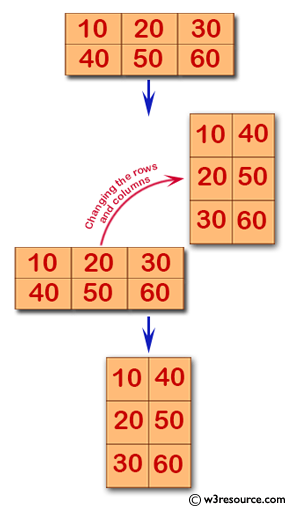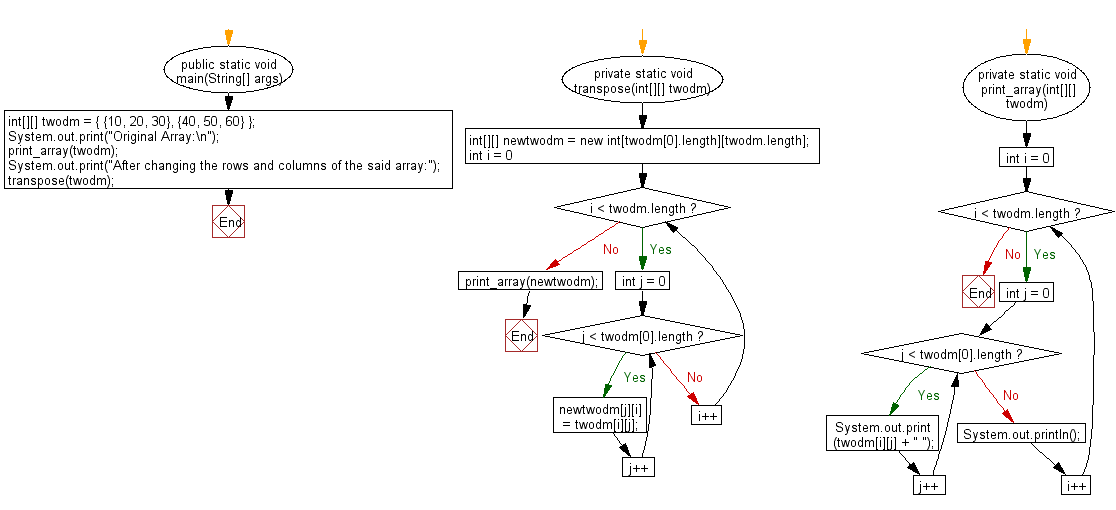﻿ Java programming exercises: Print an array after changing the rows and columns of a specified two-dimensional array - w3resource# Java Exercises: Print an array after changing the rows and columns of a specified two-dimensional array

## Java Basic: Exercise-155 with Solution

Write a Java program to print an array after changing the rows and columns of a given two-dimensional array.

Original Array:
10 20 30
40 50 60
After changing the rows and columns of the said array:10 40
20 50
30 60

Pictorial Presentation:Sample Solution:

Java Code:

``````import java.util.Scanner;
public class Solution {
public static void main(String[] args) {
int[][] twodm = {
{10, 20, 30},
{40, 50, 60}
};
System.out.print("Original Array:\n");
print_array(twodm);
System.out.print("After changing the rows and columns of the said array:");
transpose(twodm);
}

private static void transpose(int[][] twodm) {

int[][] newtwodm = new int[twodm.length][twodm.length];

for (int i = 0; i < twodm.length; i++) {
for (int j = 0; j < twodm.length; j++) {
newtwodm[j][i] = twodm[i][j];
}
}

print_array(newtwodm);
}
private static void print_array(int[][] twodm) {
for (int i = 0; i < twodm.length; i++) {
for (int j = 0; j < twodm.length; j++) {
System.out.print(twodm[i][j] + " ");
}
System.out.println();
}

}
}
```
```

Sample Output:

```Original Array:
10 20 30
40 50 60
After changing the rows and columns of the said array:10 40
20 50
30 60
```

Flowchart:Java Code Editor:

What is the difficulty level of this exercise?

﻿

## Java: Tips of the Day

Parsing dates:

```import java.io.*;
import java.util.*;
import java.text.*;

String s = "2001/09/23 14:39";

SimpleDateFormat formatter = new SimpleDateFormat ("yyyy/MM/dd H:mm");
Date d = formatter.parse(s, new ParsePosition(0));
```